# Queue input stream of calls

A random process given in some form or other and describing the appearance of calls in the queue. The input stream is usually defined by random sequences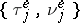, where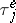,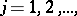are the time intervals between the arrivals in the system of the batches of requests of sizesrespectively. If, the stream is called single. Equivalently, the input stream can be given by a point process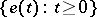, giving the number of calls arriving in the system up to time. It can be assumed, for the sake of being specific, that.

The most common requirement usually placed on the input stream is stationarity. This condition can be of two kinds: either stationarity in the narrow sense is required of the sequence(denoted by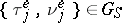), or it is required that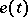be a process with increments that are stationary in the narrow sense (denoted by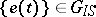). These requirements, in general, do not coincide (cf. Stationary stochastic process).

The intensity of a stationary stream is the number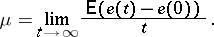If, then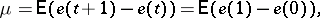so thatis equal to the mean number of calls which arrive in the system in unit time. If the sequence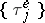is stationary and ergodic and if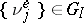, then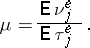In other cases the relation betweenand the distribution of the sequencecan be more complicated.

Let there be given a processwith intensityand initial value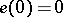. Closely associated with the numberis another parameter of the input stream, defined by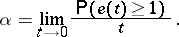This limit always exists and. If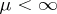, then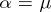if and only if the input stream is single.

In studying the properties of the input stream one often uses the so-called Palm functions(1)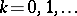(here), which have the meaning of the conditional probabilities thatcalls arrive in the interval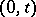under the condition that a call has arrived at time. The function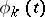is related to the distribution ofby: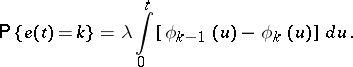If, then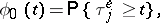A significant role in queueing theory is played by the so-called simplest, or Poisson, input stream — a stationary input stream for which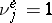,. In order to define the simplest stream in terms ofit is required that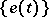be Poisson (cf. Poisson process). The increments of this process in disjoint time intervals are independent and have Poisson distributions with parameters proportional to the length of the intervals.

Non-homogeneous Poisson streams also find broad application (particularly in telephony); these are characterized as processeswith independent increments distributed by the Poisson law: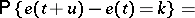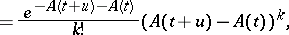where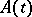is the drift function of the process (in the homogeneous case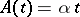).

The special role of Poisson processes in queueing theory is explained in many respects by the fundamental limit theorem for input streams, which asserts that under broad assumptions the sum of a large number of arbitrary independent stationary input streams of low intensity converges to a Poisson process. The frequent use of the assumption that the input flow is Poisson rests on the fact that in many applications real input streams are formed in precisely that way (for example, the stream of calls arriving at a telephone exchange is the sum of the weak streams originating from the individual subscribers).

Below, the fundamental limit theorem is given in two forms. The first relates to the sum of arbitrary (non-stationary) input streams.

Suppose one is given increasing sets of independent process,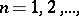depending on(that is, a triangular array), and the following notation: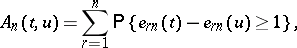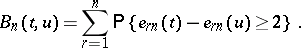In addition, for any fixed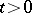, letas, uniformly in(this is the condition of low intensity of the streams). Then in order that the finite-dimensional distributions of the process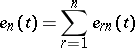converge to the distributions of a Poisson process with drift function, it is necessary and sufficient that as,If in the triangular array under discussion the processes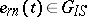and are single, then the following result also holds. Letbe the intensity ofand, as, letThen for the convergence of the finite-dimensional distributions of the processesto a distribution of a Poisson processwith parameterit is necessary and sufficient that for each,(2)

where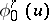is the Palm function for the process, defined by (1). If, as,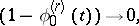uniformly in, then (2) is obviously satisfied.

For references see Queueing theory.

How to Cite This Entry:
Queue input stream of calls. A.A. Borovkov (originator), Encyclopedia of Mathematics. URL: http://www.encyclopediaofmath.org/index.php?title=Queue_input_stream_of_calls&oldid=12417
This text originally appeared in Encyclopedia of Mathematics - ISBN 1402006098